# EMBL Nano Fluid Flow Along Riga Plate in a Rotating System

EMBL Nano Fluid Flow Along Riga Plate in a Rotating System
Mathematics Discipline, Science, Engineering and Technology School, Khulna University, Khulna-9208, Bangladesh

Corresponding Author Email:
mehetajparvine@gmail.com
Page:
87-98
|
DOI:
https://doi.org/10.18280/mmc_b.882-407
25 April 2019
|
Accepted:
13 September 2019
|
Published:
30 December 2019
| Citation

OPEN ACCESS

Abstract:

This numerical investigation is related with the study of the influence of electromagnetic field for Nano fluid flow along the Riga plate in a rotating system. By using usual transformation, the systems of governing nonlinear coupled partial differential equations are transformed into dimensionless nonlinear coupled partial differential equations and these obtained equations have been solved by implicit finite difference method. The stability analysis of the dimensionless nonlinear coupled partial differential equations has been established and convergence criteria have also been analyzed. The Compaq visual FORTRAN 6.6a and MATLAB R2015a code have been used as tools to simulate the above mentioned dimensionless nonlinear coupled partial differential equations. The obtained results have been shown graphically. Also, Mesh sensitivity test, time sensitivity test (steady state solutions), validation test (comparison) have been shown graphically as well as mathematically.

Keywords:

EMF Riga plate, nano fluid, rotating system

1. Introduction

Nano fluid technology has been received a lot of attention as research topic and some important part for further development of higher performance due to the effective application in the field of electrical engineering, transportation, biomedical research etc. The Nano fluid has a higher thermal conductivity that shows significant enhancement due to the rate of heat transfer in industrial applications. Choi  was the first author who proposed the Nano fluid term and these fluid have noble properties. Haque and Alam,  has investigated numerically the transient heat and mass transfer by mixed convection flow from a vertical porous plate with induced magnetic field, constant heat and mass fluxes. Haque, Z. and Alam  has studied micropolar fluid behaviors on unsteady MHD heat and Mass transfer flow with constant heat and mass fluxes, joule heating and viscous dissipation. Also, Parven and Alam  have analyzed as well as investigated fluid flow through a parallel plates in the presence of hall current with inclined magnetic field in a rotating system.

Srait et al.  has developed a analysis about temperature dependence of thermal conductivity enhancement for Nano fluids. Unsteady convection flow of some Nano fluids past a moving vertical flat plate with heat transfer analyzed by Turkyilmazoglu . Khan and Pop  has investigated free convection boundary layer flow past a horizontal flat Plate embedded in a porous medium filled with a Nano fluid.

About five decades ago Gailitis and Lielausis  devised a Riga plate (Figure 1) which was an ingenious way to produce the wall parallel Lorentz force to control a fluid flow. This Riga plate play an important role in submarine and sheep industry. Further the Grinberg et al.  introduced the Grinberg term in contrast to the Hartmann number. Magyari and Pantokratoras  contributed a lot in investigating an aiding and opposing effects of the Lorentz force over a Riga plate with a viscous fluid flow. Hayat et al.  studied boundary layer flow of Nano fluid induced by a Riga plate with variable thickness. Adeel et al.  has investigated the steady flow of Nano fluid past Riga plate numerically. Entropy generation on viscous Nano fluid through a horizontal Riga plate for suction case has been examined by Abbas et al. . This paper discussed numerical solution for Riga plate with suction velocity.

The aim of this paper is to study the unsteady one dimensional Electromagnetic boundary layer flow of a nano fluid flow along a Riga plate with suction velocity. The governing equations of the problem have been transformed by usual transformation into a non-dimensional system of partial coupled differential equations. The implicit finite difference technique has been used to solve the dimensionless system of non-linear partial differential equations. The obtained results have been shown graphically.

2. Mathematical Model of the Flow

Consider the electromagnetic hydrodynamic mixed-convection boundary-layer flow of a Nano fluid with weakly conducting based fluid induced by a Riga-plate placed at y=0 with x-axis aligned vertically upward. Due to the plate position, $\frac{\partial u}{\partial x}=0$ and the continuity equation $\frac{\partial u}{\partial x}+\frac{\partial v}{\partial y}=0$ gives that $\frac{\partial v}{\partial y}=0$, thus the y component of the velocity is constant (v=v0.) Thus, the fluid velocity is given as q=u(y,t)i+vj+w(y,t)k. The plate with suction velocity v0 is moving with constant velocity in x -direction in a viscous based Nano fluid. The Riga-plate consists of an alternating array of electrodes and permanent magnets mounted on a plane surface (see Figure 2). At sheet temperature T and the nano particle fraction ϕ takes the constant values Tw and ϕw respectively. The ambient values of T and ϕ are given as T and ϕ.

## 1.png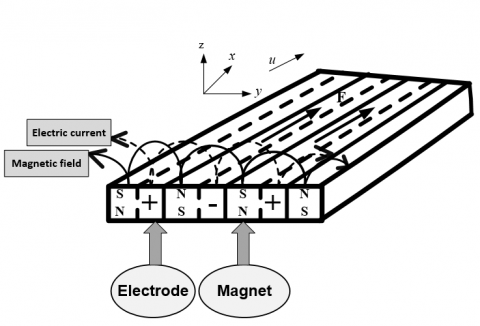Figure 1. Physical representation of the Riga Plate

## 2.png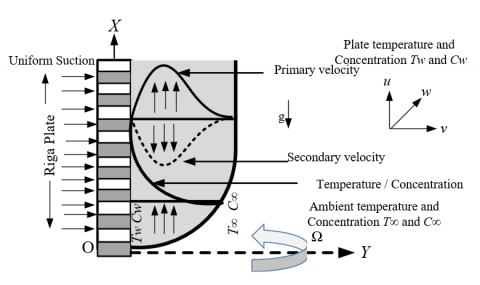Figure 2. Geometrical configuration and coordinate system

Employing the Oberbeck–Boussinesq approximation and the assumption that the nano particle concentration is diluted, the boundary layer equations are related to the unsteady one dimensional problem governed by the following system of coupled non-linear partial differential equations which are given as follows:

Continuity equation

v=Constant=-v0          (1)

Momentum equations

$\frac{\partial u}{\partial \tau }-{{v}_{0}}\frac{\partial u}{\partial y}=\upsilon \frac{{{\partial }^{2}}u}{\partial {{y}^{2}}}+(1-{{\phi }_{\infty }})g{{\beta }^{*}}(T-{{T}_{\infty }})-\frac{{{\rho }_{p}}-{{\rho }_{f}}}{{{\rho }_{f}}}g(\phi -{{\phi }_{\infty }})+\frac{\pi {{J}_{0}}{{M}_{0}}}{8{{\rho }_{f}}}\exp (-\frac{\pi }{a}y)+2\Omega w$                     (2)

$\frac{\partial w}{\partial \tau }-{{v}_{0}}\frac{\partial w}{\partial y}=\upsilon \frac{{{\partial }^{2}}w}{\partial {{y}^{2}}}+\frac{\pi {{J}_{0}}{{M}_{0}}}{8{{\rho }_{f}}}\exp (-\frac{\pi }{a}y)-2\Omega u$                                                             (3)

The equation of conservation of energy

$\frac{\partial T}{\partial \tau }-{{v}_{0}}\frac{\partial T}{\partial Y}=\alpha \left( \frac{{{\partial }^{2}}T}{\partial {{Y}^{2}}} \right)+\tau \left[ {{D}_{B}}\left[ \left( \frac{\partial C}{\partial y}\cdot \frac{\partial T}{\partial y} \right) \right]+\frac{{{D}_{T}}}{{{T}_{\infty }}}\left[ {{\left( \frac{\partial T}{\partial y} \right)}^{2}} \right] \right]$                (4)

The equation of Concentration

$\frac{\partial C}{\partial \tau }-{{v}_{0}}\frac{\partial C}{\partial y}={{D}_{B}}\left( \frac{{{\partial }^{2}}C}{\partial {{y}^{2}}} \right)+\frac{{{D}_{T}}}{{{T}_{\infty }}}\left( \frac{{{\partial }^{2}}T}{\partial {{y}^{2}}} \right)$         (5)

With their respective boundary conditions are as follows:

\begin{align} & u=0\,,\ \ w=0,\,\ \ \ \ T=1,\,\,\ \ \ \,C=1\,\,\ \ \ \ \ \ \ \ \,at\,\,Y=0 \\ & u\to 0\,,\,w=0\ \ \ \,\ \ \ T=0,\,\ \ \ \ C=0\,\,\ \ \ \ \ \ \ as\,\,\,\,Y\to \infty \\ \end{align}        (6)

3. Solution

It is required to make the equations (1) to (5) into the dimensionless equations. The solution of the governing equations (1) to (5) under the boundary conditions (6) are based on the finite difference method. The following dimensionless variables have been used for obtaining the dimensionless governing equations.

\begin{align} & X=\frac{x}{l},Y=\frac{y}{L},U=\frac{u}{{{U}_{w}}},w=\frac{W}{{{U}_{w}}},\theta =\frac{T-{{T}_{\infty }}}{{{T}_{w}}-{{T}_{\infty }}}, \\ & \phi =\frac{C-{{C}_{\infty }}}{{{C}_{w}}-{{C}_{\infty }}},L=\frac{a}{\pi },l=\frac{{{U}_{w}}{{L}^{2}}}{\upsilon },\tau =\frac{t{{U}_{w}}}{l} \\ \end{align}          (7)

The obtained dimensionless governing non-linear coupled partial differential equations are given as follows:

$\frac{\partial V}{\partial Y}\,\,=0$        (8)

$\frac{\partial U}{\partial \tau }-S\frac{\partial U}{\partial Y}=\frac{{{\partial }^{2}}U}{\partial {{Y}^{2}}}+{{R}_{c}}\theta -{{N}_{r}}\phi +{{H}_{a}}{{e}^{-Y}}+RW$                 (9)

$\frac{\partial W}{\partial \tau }-S\frac{\partial W}{\partial Y}=\frac{{{\partial }^{2}}W}{\partial {{Y}^{2}}}+{{H}_{a}}{{e}^{-Y}}-RU$           (10)

$\frac{\partial \theta }{\partial \tau }-S\frac{\partial \theta }{\partial Y}=\frac{1}{{{p}_{r}}}\frac{{{\partial }^{2}}\theta }{\partial {{Y}^{2}}}+{{N}_{b}}\frac{\partial \theta }{\partial Y}\frac{\partial \phi }{\partial Y}+{{N}_{t}}{{(\frac{\partial \theta }{\partial Y})}^{2}}$               (11)

$\frac{\partial \phi }{\partial \tau }-S\frac{\partial \phi }{\partial Y}=\frac{1}{{{L}_{e}}}\frac{{{\partial }^{2}}\phi }{\partial {{Y}^{2}}}+\frac{1}{{{L}_{e}}}\frac{{{N}_{t}}}{{{N}_{b}}}\frac{{{\partial }^{2}}\theta }{\partial {{Y}^{2}}}$                  (12)

The dimensionless boundary conditions are given as follows:

\begin{align} & U=0\,,\ \ W=0,\,\ \ \ \ T=1,\,\,\ \ \ \,C=1\,\,\ \ \ \ \ \ \ \ \,at\,\,Y=0 \\ & U\to 0\,,\,\ W=0\ \ \,\ \ \ T=0,\,\ \ \ \ C=0\,\,\ \ \ \ \ \ \ as\,\,\,\,Y\to \infty \\ \end{align}                  (13)

The non-dimensional parameters are given as follows:

Richardson number: ${{R}_{c}}=\frac{{{a}^{2}}\Delta T}{{{\pi }^{2}}\nu {{U}_{w}}}\left( 1-{{\phi }_{\infty }} \right)g{{\beta }^{*}}$, Nanoparticle concentration flux: ${{N}_{r}}=\frac{{{a}^{2}}\Delta \phi }{{{\pi }^{2}}\nu {{U}_{w}}}\frac{{{\rho }_{p}}-{{\rho }_{f}}}{{{\rho }_{f}}}$, Hartmann number: ${{H}_{a}}=\frac{1}{8\pi }\frac{{{a}^{2}}{{J}_{0}}{{M}_{0}}}{v{{U}_{w}}{{\rho }_{f}}}$, Brownian motion parameter: ${{N}_{b}}=\frac{\tau {{D}_{B}}\Delta \phi }{v}$,Lewis Number: ${{L}_{e}}=\frac{v}{{{D}_{B}}}$, Thermophoresis parameter: ${{N}_{t}}=\frac{\tau {{D}_{T}}\Delta T}{v{{T}_{\infty }}}$, Kinematic viscosity: $v=\frac{\mu }{{{\rho }_{f}}}$, Prandtl Number: ${{p}_{r}}=\frac{v}{a}$, Rotating parameter: $R=\frac{2{{a}^{2}}\Omega }{{{\pi }^{2}}\upsilon }$, Suction parameter: $S=\frac{{{v}_{0}}}{{{U}_{\infty }}}$.

3.1 Shear stress, Nusselt and Sherwood number

The quantities of chief physical interest are Shear Stress, Nusselt number, and Sherwood number. From the Primary velocity field, the effects of various parameters on the local shear stress has been investigated. The following equations represent the local shear stress at the plate.

Local shear stress,

${{\tau }_{ul}}={{R}_{{}}}{{\left( \frac{\partial U}{\partial y} \right)}_{y=0}}$                      (14)

and which are proportional to ${{\left( \frac{\partial U}{\partial y} \right)}_{y=0}}$

From the secondary velocity field, the effects of various parameters on the local shear stress have been formulated. The following equations represent the local shear stress at the plate.

Local shear stress,

${{\tau }_{wl}}={{R}_{{}}}{{\left( \frac{\partial W}{\partial y} \right)}_{y=0}}$           (15)

and which are proportional to ${{\left( \frac{\partial W}{\partial y} \right)}_{y=0}}$

From the temperature field, the effects of various parameters on the local and average heat coefficients have been formulated. The following equations represent the local and average heat transfer rate that is well known Nusselt number.

Local Nusselt number,

$N{{u}_{l}}={{\left( 1+R \right)}_{{}}}.{{\left( -\frac{\partial T}{\partial y} \right)}_{y=0}}$                          (16)

and which are proportional to ${{\left( -\frac{\partial \overline{T}}{\partial Y} \right)}_{y=0}}$

And from the concentration field, the effects of various parameter on the local mass transfer coefficients has analyzed. The following represent the local and average mass transfer rate that is well known Local Sherwood number,

$S{{h}_{l}}=.{{\left( -\frac{\partial C}{\partial y} \right)}_{y=0}}$             (17)

and which are proportional to ${{\left( -\frac{\partial \overline{C}}{\partial Y} \right)}_{y=0}}$

3.2 Numerical technique

To solve the dimensionless system (8) to (13) by the implicit finite difference method subject to the boundary conditions, a set of the finite difference equation is required. For which, the region within the boundary layer is divided into a grid or mesh grid of lines perpendicular to Y axis where Y-axis is normal to the plate is as shown in Figure 3.

## 3.pngFigure 3. Finite difference space grid

Here it is assumed that the maximum length of the boundary layer is ${{Y}_{\max }}\left( =25 \right)$ as corresponding to$Y\to \infty$ i.e. $Y$varies from 0 to 25. The number of grid spacing $n=80$  along the $Y$directions. It is assumed that $Y$is constant mesh size $Y$directions and taken follows.

$Y=25(0\le x\le 100)$; With the smaller time-step, $\Delta \tau =0.025$

Consider  ${{U}^{'}},{{V}^{'}},{{W}^{'}},{{\theta }^{'}},{{\phi }^{'}}$ denote the values of $U,V,\theta ,\phi$ at the end of time-step respectively.

\begin{align} & \ {{\left( \frac{\partial U}{\partial \tau } \right)}_{i,j}}=\frac{{{U}^{'}}_{j}-{{U}_{j}}}{\Delta X},{{\left( \frac{\partial U}{\partial Y} \right)}_{i,j}}=\frac{{{U}_{j+1}}-{{U}_{j}}}{\Delta Y},\,{{\left( \frac{{{\partial }^{2}}U}{\partial {{Y}^{2}}} \right)}_{i,j}}=\frac{{{U}_{j+1}}-2{{U}_{j}}+{{U}_{j-1}}}{{{(\Delta Y)}^{2}}}, \\ & {{\left( \frac{\partial W}{\partial \tau } \right)}_{i,j}}=\frac{{{W}^{'}}_{j}-{{W}_{j}}}{\Delta \tau },{{\left( \frac{\partial W}{\partial Y} \right)}_{i,j}}=\frac{{{W}_{j+1}}-{{W}_{j}}}{\Delta Y},{{\left( \frac{{{\partial }^{2}}W}{\partial {{Y}^{2}}} \right)}_{i,j}}=\frac{{{W}_{j+1}}-2{{W}_{j}}+{{W}_{j-1}}}{{{(\Delta Y)}^{2}}}, \\ & {{\left( \frac{\partial \theta }{\partial \tau } \right)}_{i,j}}=\frac{{{\theta }^{'}}_{j}-{{\theta }_{j}}}{\Delta \tau },\ {{\left( \frac{\partial \theta }{\partial Y} \right)}_{i,j}}=\frac{{{\theta }_{j+1}}-{{\theta }_{j}}}{\Delta Y}\,,{{\left( \frac{{{\partial }^{2}}\theta }{\partial {{Y}^{2}}} \right)}_{i,j}}=\frac{{{\theta }_{j+1}}-2{{\theta }_{j}}+{{\theta }_{j-1}}}{\Delta {{Y}^{2}}},\ \\ & {{\left( \frac{\partial \phi }{\partial \tau } \right)}_{i,j}}=\frac{{{\phi }^{'}}_{j}-{{\phi }_{j}}}{\Delta \tau },{{\left( \frac{\partial \phi }{\partial Y} \right)}_{i,j}}=\frac{{{\phi }_{j+1}}-{{\phi }_{j}}}{\Delta Y}\ ,\ \ {{\left( \frac{{{\partial }^{2}}\phi }{\partial {{Y}^{2}}} \right)}_{i,j}}=\frac{{{\phi }_{j+1}}-2{{\phi }_{j}}+{{\phi }_{j-1}}}{\Delta {{Y}^{2}}} \\ \end{align}

Using the above implicit finite difference approximation the following appropriate set of finite difference equations are obtained as follows:

Momentum equation

$\frac{{{U}^{'}}_{j}-{{U}_{j}}}{\Delta t}-S(\frac{{{U}_{j+1}}-{{U}_{j}}}{\Delta Y})=\frac{{{U}_{j+1}}-2{{U}_{j}}+{{U}_{j-1}}}{{{(\Delta Y)}^{2}}}+{{R}_{c}}{{\theta }_{j}}-{{N}_{r}}{{\phi }_{j}}+{{H}_{a}}{{e}^{-Y}}+R{{W}_{j}}$              (18)

Momentum equation

$\frac{{{W}^{'}}_{i,j}-{{W}_{i,j}}}{\Delta t}-S(\frac{{{W}_{j+1}}-{{W}_{j}}}{\Delta Y})=\frac{{{W}_{j+1}}-2{{W}_{j}}+{{W}_{j-1}}}{{{(\Delta Y)}^{2}}}+{{H}_{a}}{{e}^{-Y}}-R{{U}_{j}}$            (19)

Energy equation

\begin{align} & \frac{{{{{\theta }'}}_{j}}-{{\theta }_{j}}}{\Delta \tau }-S(\frac{{{\theta }_{j+1}}-{{\theta }_{j}}}{\Delta Y})=\frac{1}{{{p}_{r}}}(\frac{{{\theta }_{j+1}}-2{{\theta }_{j}}+{{\theta }_{j-1}}}{{{(\Delta Y)}^{2}}}) \\ & \,\,\,\,\,\,\,\,\,\,\,\,\,\,\,\,\,\,\,\,\,\,\,\,\,\,\,\,\,\,\,\,\,\,\,\,\,\,\,\,\,\,\,\,\,\,\,\,\,\,\,\,\,\,\,\,+{{N}_{b}}(\frac{{{\theta }_{j+1}}-{{\theta }_{j}}}{\Delta Y})(\frac{{{\phi }_{j+1}}-{{\phi }_{j}}}{\Delta Y})+{{N}_{t}}{{(\frac{{{\theta }_{j+1}}-{{\theta }_{j}}}{\Delta Y})}^{2}} \\ \end{align}            (20)

Concentration equation

\begin{align} & \frac{{{{{\phi }'}}_{j}}-{{\phi }_{j}}}{\Delta \tau }+U+{{V}_{i,j}}(\frac{{{\phi }_{j+1}}-{{\phi }_{j}}}{\Delta Y})=\frac{1}{{{L}_{e}}}(\frac{{{\phi }_{j+1}}-2{{\phi }_{j}}+{{\phi }_{j-1}}}{{{(\Delta Y)}^{2}}}) \\ & \,\,\,\,\,\,\,\,\,\,\,\,\,\,\,\,\,\,\,\,\,\,\,\,\,\,\,\,\,\,\,\,\,\,\,\,\,\,\,\,\,\,\,\,\,\,\,\,\,\,\,\,\,\,\,\,\,\,\,\,\,\,\,\,+\frac{1}{{{L}_{e}}}\frac{{{N}_{t}}}{{{N}_{b}}}(\frac{{{\theta }_{j+1}}-2{{\theta }_{j}}+{{\theta }_{j-1}}}{{{(\Delta Y)}^{2}}}) \\ \end{align}         (21)

And the boundary conditions are given as follows:

\begin{align} & {{U}_{0}}^{n}=0,{{V}_{0}}^{n}=0,\,\,{{W}_{0}}^{n}=0,\,\,{{\theta }^{n}}_{0}=1,\,\,\,{{\phi }^{n}}_{0}=1\,\,\, \\ & {{U}_{L}}^{n}=0\,,\,{{W}_{L}}^{n}=0,\,\,\,{{\theta }^{n}}_{L}=0,\,\,{{\phi }^{n}}_{L}=0\,\,where\,\,L\to \infty \, \\ \end{align}          (22)

Here the subscripts$j$ designate the grid points with  $y$ coordinates respectively and the subscript $n$ represents a value of time, $\tau =n\Delta \tau$ every where. From the initial condition (22), the values of $U$$W$,$\theta$ and $\phi$are known at$\tau =0$.$U$,$W$, $\theta$ and $\phi$ should eventually converge to values which are approximately the steady state solution of equations (17) to (20).

4. Stability and Convergence Analysis

Stability conditions of the problem are given as follows:

$\frac{S\Delta \tau }{\Delta Y}+\frac{2}{{{p}_{r}}}\frac{\Delta \tau }{{{(\Delta Y)}^{2}}}+2{{N}_{b}}\phi \frac{\Delta \tau }{{{(\Delta Y)}^{2}}}+2{{N}_{t}}\theta \frac{\Delta \tau }{{{(\Delta Y)}^{2}}}\le 1$

$\frac{1}{{{L}_{e}}}\frac{2\Delta \tau }{{{(\Delta Y)}^{2}}}-\frac{S\Delta \tau }{\Delta Y}\le 1$

Using $\Delta Y=0.3125,\Delta \tau =0.025$ and the initial conditions, the above equations are given as follows:

${{L}_{e}}\ge -0.372,S\le 21.8$

5. Results and Discussion

Due to analyze the physical situation of the model, the steady-state numerical values of the non-dimensional primary velocity (U), secondary velocity (W), temperature (θ) and concentration (ϕ) distributions within the boundary layer have been computed. Also, the effect on local shear stress (Primary and secondary), local Nusselt and local Sherwood number for different values of Brownian motion parameter Nb, Thermophoresis parameter Nt, Lewis number Le, Richardson number Rc, Suction parameter S and Rotation parameter R have been shown graphically.

5.1 Mesh sensitivity and code validation tests

To verify the effects of grid space for n, the computations have been carried out for three different grid spaices such as n=60; n=80 and n=100 are shown in Figure 4(a). But negligible changes have been seen among these graphs. According to this situation, the results of primary velocity, secondary velocity, temperature and distributions have been carried out for n=80. The computations have been carried out by using MATLAB R2015a code and Studio Developer FORTRAN 6.6a (SDF) code. The results of both codes are qualitatively same but not and quantitatively which are shown in Figures 4(b,c). From these figures, the same results have been occurred on primary velocity for different values of suction parameters which have been shown for the results of both codes.

5.2 Time sensitivity test (steady state solution)

To obtain the steady-state solution, the computations have been carried out for different time step sizes such as τ=5.0, 10.0, 20.0, 40.0, 50.0, and 60.0. It is observed that the result of computations for different time distributions, however, shows little changes after τ=20.0. Thus the solutions for τ=40.0 are taken essentially as the steady-state solutions. The time variation for primary (U), secondary velocity (W), temperature (θ) and concentration (ϕ) distributions are shown in Figures 5 to 6.

5.3 Validation test (comparison)

A qualitative and quantitative comparisons of the present steady-state results with the published results of Abaas et al.  are presented in Figure 7. Thus, the present results and the published results of Abaas et al.  are qualitatively quite same.

Figure $7(\mathrm{a})$ The effect of Brownian motion $N_{b}$ parameter and Prandtl's number $p_{r}$ on Concentration distribution of the present study without Rotation parameter. And (b) The effect of Brownian motion $N_{b}$ parameter and Prandtl's number $p_{r}$ on Concentration distribution of Abbas et al. .

5.4 Effects of different parameters on velocity distribution, temperature distribution, and concentration distribution

In order to get the clear concept of physical properties of the problem, the effects of different parameters namely Rotation parameter R, Richardson parameter Rc, Suction parameter S, Brownian motion parameter Nb, Thermophoresis parameter Nt, Lewis number Leare presented graphically. For numerical computations, it has been used S=2.00 Nr=0.30, Rc=1.00, R=0.10, Ha=1.00, Nt=0.08, Nb=0.10, Le=1.10, pr=1.00 arbitrarily.

The effects of Rotation parameter R on Primary velocity is shown in Figure 8. It is observed from Figure 8 that the primary velocity decreases with the increase of the Rotation parameter R. On the other hand secondary velocity increases with the increase of the Rotation parameter R which are shown in Figure 9. It is observed from Figure 10 that the primary velocity increases with the increase of Richardson parameter Rcwhile secondary velocity decreases with the increase of Richardson parameter Rc which are shown in Figure 11. Also, it is seen from Figure 10 and Figure 11 that the boundary layer growth of primary velocity is weaker than secondary velocity.

The effects of Suction parameter S on primary velocity are shown in Figure 12. It is observed that primary velocity decreases with the decrease of Suction parameter S. The Figure 13 displays that the secondary velocity increases with the decrease of Suction parameter S.

Figure 14 shows that temperature distribution for different values of Suction parameter S, the temperature gradually decreases with the increase of Suction parameter S.

The effects of Brownian motion parameter Nb on Temperatur distribution increases in Figure 15. It is observed that temperature distribution increases with the increase of Brownian motion parameter Nb

The effects of Suction parameter S on Concentration distribution decreases in Figure 16. It is observed from this figure that Concentration distribution decreases with the increase of Suction parameter S.

In Figure 17, Concentration distribution is decreases with the decrease of Brownian motion parameter Nb. Figure 18 displays the effects of Thermophoresis parameter Nt on Concentration distribution. It is seen that Concentration distribution increases with the increase of Thermophoresis motion parameter Nt. Figure 19 shows the concentration distribution for different values of Lewis number Le, while Concentration distribution decreases with the increase of Lewis number Le.

## 4.png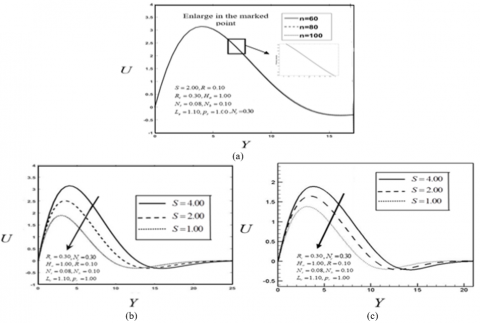Figure 4. Justification of grid space for (a) primary velocity (MATLAB R2015a). Effect of Suction parameter S for (b) primary velocity (MATLAB R2015a) and (c) primary velocity (Studio Developer FORTRAN 6.6a)

## 5.png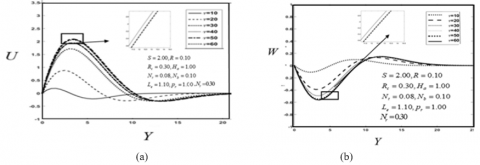Figure 5. Illustration of time variation for (a) primary velocity and (b) secondary velocity

## 6.png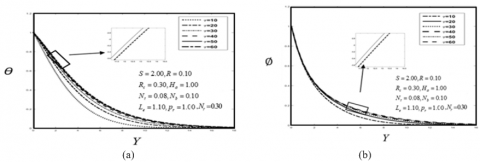Figure 6. Illustration of time variation for (a) Temperature distribution and (b) Concentration distribution

## 7.png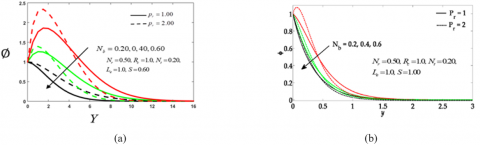Figure 7. Comparison between steady-state results and the published results

## 8.png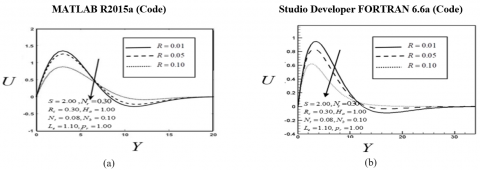Figure 8. Effect of Rotation parameter R for (a) primary velocity (MATLAB R2015a) and (b) Primary velocity (Studio Developer FORTRAN 6.6a)

## 9.png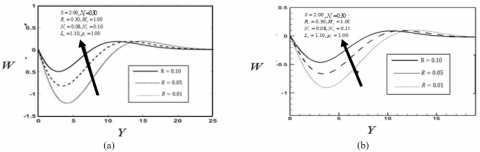Figure 9. Effect of Rotation parameter R for (a) Secondary velocity (MATLAB R2015a) and (b) Secondary velocity (Studio Developer FORTRAN 6.6a)

## 10.pngFigure 10. Effect of Richardson number Rc for (a) primary velocity (MATLAB R2015a) and (b) Primary velocity (Studio Developer FORTRAN 6.6a)

## 11.png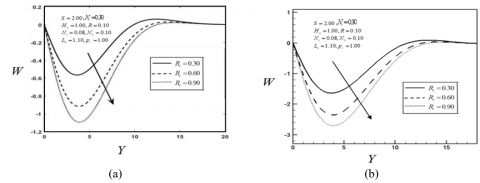Figure 11. Effect of Richardson number Rc for (a) Secondary velocity (MATLAB R2015a) and (b) Secondary velocity (Studio Developer FORTRAN 6.6a)

## 12.png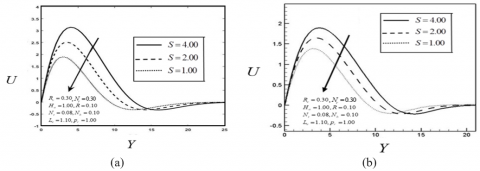Figure 12. Effect of Suction parameter S for (a) primary velocity (MATLAB R2015a) and (b) Primary velocity (Studio Developer FORTRAN 6.6a)

## 13.pngFigure 13. Effect of Suction parameter S for (a) Secondary velocity (MATLAB R2015a) and (b) Secondary velocity (Studio Developer FORTRAN 6.6a)

## 14.png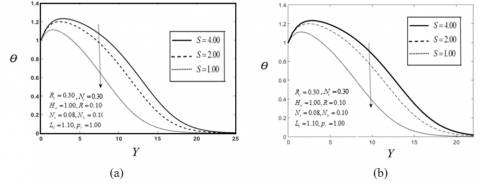Figure 14. Effect of Suction parameter S for (a) Temperature distribution (MATLAB R2015a) and Temperature distribution (Studio Developer FORTRAN 6.6a)

## 15.png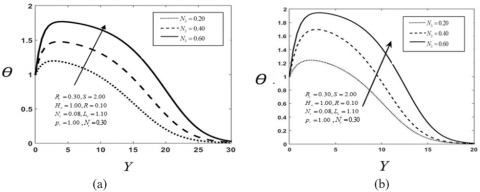Figure 15. Effect of Brownian motion parameter Nb for (a) Temperature distribution (MATLAB R2015a) and (b) Temperature distribution (Studio Developer FORTRAN 6.6a)

## 16.png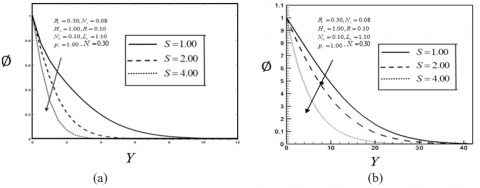Figure 16. Effect of Suction parameter S for (a) Concentration distribution (MATLAB R2015a) and (b) Concentration distribution (Studio Developer FORTRAN 6.6a)

## 17.png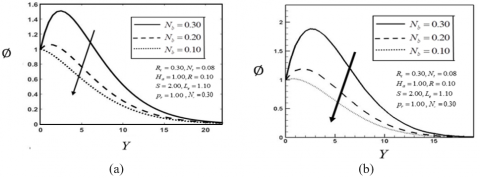Figure 17. Effect of Brownian motion parameter Nb for (a) Concentration distribution (MATLAB R2015a) and (b) Concentration distribution (Studio Developer FORTRAN 6.6a)

## 18.png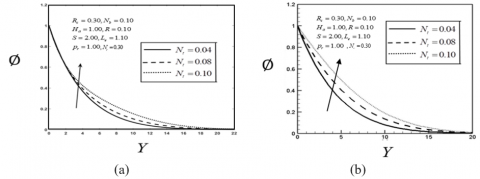Figure 18. Effect of Thermophoresis parameter Nt for (a) Concentration distribution (MATLAB R2015a) and (b) Concentration distribution (Studio Developer FORTRAN 6.6a)

## 19.pngFigure 19. Effect of Lewis number Le for (a) Concentration distribution (MATLAB R2015a) and (b)Concentration distribution (Studio Developer FORTRAN 6.6a)

5.5 Effects of different parameters on local shear stress, local Nusselt number, and local Sherwood number

It is observed from Figs. 20 and 21 that the effects of Rotation parameter $R$on primary local shear stress  decreases gradually for decreasing the values of Rotation parameter $R$ while the effects of Rotation parameter $R$on Secondary local shear stress  increases for decreasing the values of Rotation parameter $R$. It is seen from the figures, the primary local shear stress have strong decreasing effect while the secondary local shear stress have weak increasing effect.

The effects of Richardson number ${{R}_{c}}$on Primary and secondary local shear stress are shown in Figs22 and 23. From the figures it is observed that primary local shear stress  increases with the increase of Richardson number ${{R}_{c}}$ while the Secondary local shear stress  decreases with the increase of Richardson number ${{R}_{c}}$. It is seen from the figures, the secondary local shear stress have strong decreasing effect while the primary local shear stress have weak increasing effect. The effects of Suction parameter $S$ on Primary and secondary local shear stress are shown in Figs24 and 25. From these figures it is observed that primary local shear stress decreases with the decrease of the Suction parameter $S$while the Secondary velocity, secondary local shear stress increases with the increase of Suction parameter $S$. It is seen from the figures, the secondary local shear stress have strong decreasing effect while the primary local shear stress have weak increasing effect.

It is observed from Fig. 26 that the effect of Brownian motion parameter ${{N}_{b}}$on local Nusselt number  increases with the increase of Brownian motion parameter ${{N}_{b}}$. The effect of Thermoporasis motion parameter ${{N}_{t}}$ on the local Nusselt number  decreases with the increase of Thermoporasis motion parameter ${{N}_{t}}$which is shown in Fig. 27. The effect of Suction parameter  $S$ on Sherwood number decreases for increasing  the values of Suction parameter  $S$.

## 20.png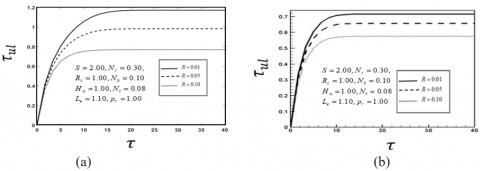Figure 20. Effect of Rotation parameter R for (a) primary local shear stress (MATLAB R2015a) and (b) Primary local shear stress (Studio Developer FORTRAN 6.6a)

## 21.png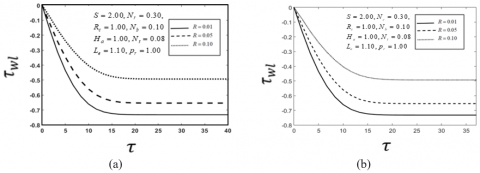Figure 21. Effect of Rotation parameter R for (a)) Secondary local shear stress (MATLAB R2015a) and (b) Secondary local shear stress (Studio Developer FORTRAN 6.6a)

## 22.png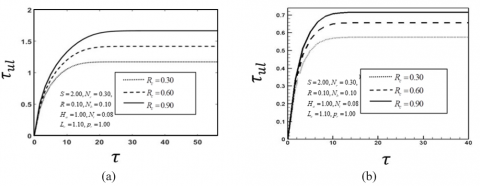Figure 22. Effect of Richardson number Rc for (a) Primary local shear stress (MATLAB R2015a) and (b) Primary local shear stress (Studio Developer FORTRAN 6.6a)

## 23.pngFigure 23. Effect of Richardson number Rc for (a) Secondary local shear stress (MATLAB R2015a) and (b) Secondary local shear stress (Studio Developer FORTRAN 6.6a)

## 24.pngFigure 24. Effect of Suction parameter S for (a) Primary local shear stress (MATLAB R2015a) and (b) Primary local shear stress (Studio Developer FORTRAN 6.6a)

## 25.png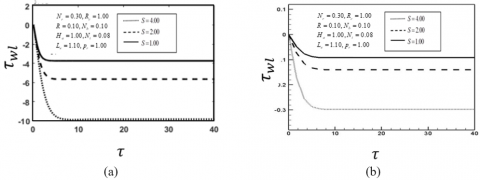Figure 25. Effect of Suction parameter S for (a) Secondary local shear stress (MATLAB R2015a) and (b)Secondary local shear stress (Studio Developer FORTRAN 6.6a)

## 26.pngFigure 26. Effect of Browninan motion parameter Nb for (a) local Nusselt number (MATLAB R2015a) and (b) local Nusselt number (Studio Developer FORTRAN 6.6a)

## 27.png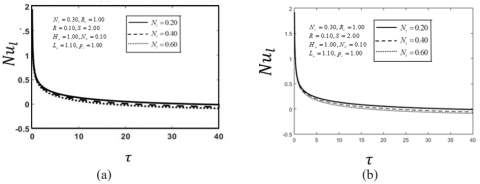Figure 27. Effect of Thermophoresis parameter Nt for (a) local Nusselt number (MATLAB R2015a) and (b) local Nusselt number (Studio Developer FORTRAN 6.6a)

## 28.pngFigure 28. Effect of Suction parameter S for (a) local shearwood number (MATLAB R2015a) and (b) local shearwood number (Studio Developer FORTRAN 6.6a)

6. Conclusions

The solution of one dimensional unsteady viscous incompressible nanofluid flow through Riga plate with rotation has been investigated. The obtained results have been discussed for different values of important parameters. The physical properties are shown graphically for different values of corresponding parameters. Among some important findings of this investigation are mentioned here;

(1) The primary velocity, primary local shear stress decrease with the increase of Rotation parameter R while the secondary velocity, secondary local shear stress increase with the increase of Rotation parameter R.

(2) The primary velocity, Primary local shear stress increase with the increase of Richardson parameter Rcwhile the secondary velocity, secondary local shear stress decrease with the increase of Richardson parameter Rc.

(3) The primary velocity, Primary local shear stress decrease with the decrease of the Suction parameter S as well as Secondary velocity, secondary local shear stress increase with the increase of Suction parameter S.

(4) The temperature distribution, the local Nusselt number increase with the increase of Brownian motion parameter Nb

(5) The temperature distribution, the local Nusselt number decrease with the decrease of Thermophoresis parameter Nt.

(6) The concentration distribution, the local Sherwood number decrease with the increase of Suction parameter S.

Nomenclature
 B Magnetic induction ν Kinematic viscosity of the fluid C Nanoparticle concentration μ Dynamic viscosity of the fluid Cw Nanoparticle Concentration at Riga plate α Thermal diffusivity C∞ Ambient nanoparticle Concentration σ Conductivity of the material cp Specific heat capacity ρf Fluid density DB Brownian diffusion coefficient θ Dimensionless Temperature DT Thermophoresis diffusion coefficient Rc Richardson number K Thermal conductivity Ha Hartman number T Fluid Temperature Nr Nanoparticle Concentration flux parameter Tw Temperature at the surface of the Riga plate Pr Prandtl number Nb Brownian motion parameter t Time Nt Thermophoresis parameter u,v,w Velocity components along x, y andz-axes respectively Le Lewis number U,V,W Dimensionless velocity components R Rotating parameter T∞ Ambient Temperature as y tends to infinity S Suction parameter x' Dimensionless axial distance along plate W Surface condition y' Dimensionless normal distance due to plate ∞ Condition far away from the surface
References

 Choi, S.U., Eastman, J.A. (1995). Enhancing thermal conductivity of fluids with nanoparticles (No. ANL/MSD/CP-84938; CONF-951135-29). Argonne National Lab., IL (United States).

 Haque, M.M., Mahmud Alam, M.D. (2009). Transient heat and mass transfer by mixed convection flow from a vertical porous plate with induced magnetic field, constant heat and mass fluxes. AMSE Journals, Modelling B, Measurement and Control, Mechanics and Thermics, 78: 3-4.

 Haque, Z., Alam, M.M. (2011). Micropolar fluid behaviours on unsteady MHD heat and Mass transfer flow with constant heat and mass fluxes, joule heating and viscous dissipation. AMSE Journal, 80(2).

 Perven, R., Alam, M.M. (2015). Fluid flow through parallel plates in the presence of hall current with inclined magnetic field in a rotating system. AMSE Journals–Series: Modelling B, 84(1): 49-68.

 Das, S.K., Putra, N., Thiesen, P., Roetzel, W. (2003). Temperature Dependence of Thermal Conductivity Enhancement for Nanofluids. In the Proceedings of the ASME Int. Mech.Eng.Cong.and Exp., San Francisco, USA, ASME, FED 231/MD, 125(4): 567-574. https://doi.org/10.1115/1.1571080

 Turkyilmazoglu, M. (2014). Unsteady convection flow of some nanofluids past a moving vertical flat plate with heat transfer. Journal of heat transfer, 136(3). https://doi.org/10.1115/1.4025730

 Khan, W.A., Pop, I. (2011). Free convection boundary layer flow past a horizontal flat plate embedded in a porous medium filled with a nanofluid. Journal of Heat Transfer, 133(9). https://doi.org/10.1115/1.4003834

 Lielausis, O. (1961). On a possibility to reduce the hydrodynamic resistance of a plate in an electrolyte. Appl. Magnetohydrodyn., 12: 143-146.

 Grinberg, E. (1961). On determination of properties of some potential fields. Applied Magnetohydrodynamics. Reports of the Physics Institute, 12: 147-154.

 Magyari, Pantokratoras, (2011). The Blasius and Sakiadis flow along a Riga-plate. Journal of Progress in Computational Fluid Dynamics, 11(5): 329–333.

 Hayat, T., Abbas, T., Ayub, M., Farooq, M., Alsaedi, A. (2016). Flow of nanofluid due to convectively heated Riga plate with variable thickness. Journal of Molecular Liquids, 222: 854-862. https://doi.org/10.1016/j.molliq.2016.07.111

 Ahmad, A., Asghar, S., Afzal, S. (2016). Flow of nanofluid past a Riga plate. Journal of Magnetism and Magnetic materials, 402: 44-48. https://doi.org/10.1016/j.jmmm.2015.11.043

 Abbas, T., Ayub, M., Bhatti, M.M., Rashidi, M.M., Ali, M.E.S. (2016). Entropy generation on nanofluid flow through a horizontal Riga plate. Entropy, 18(6): 223. https://doi.org/10.3390/e18060223